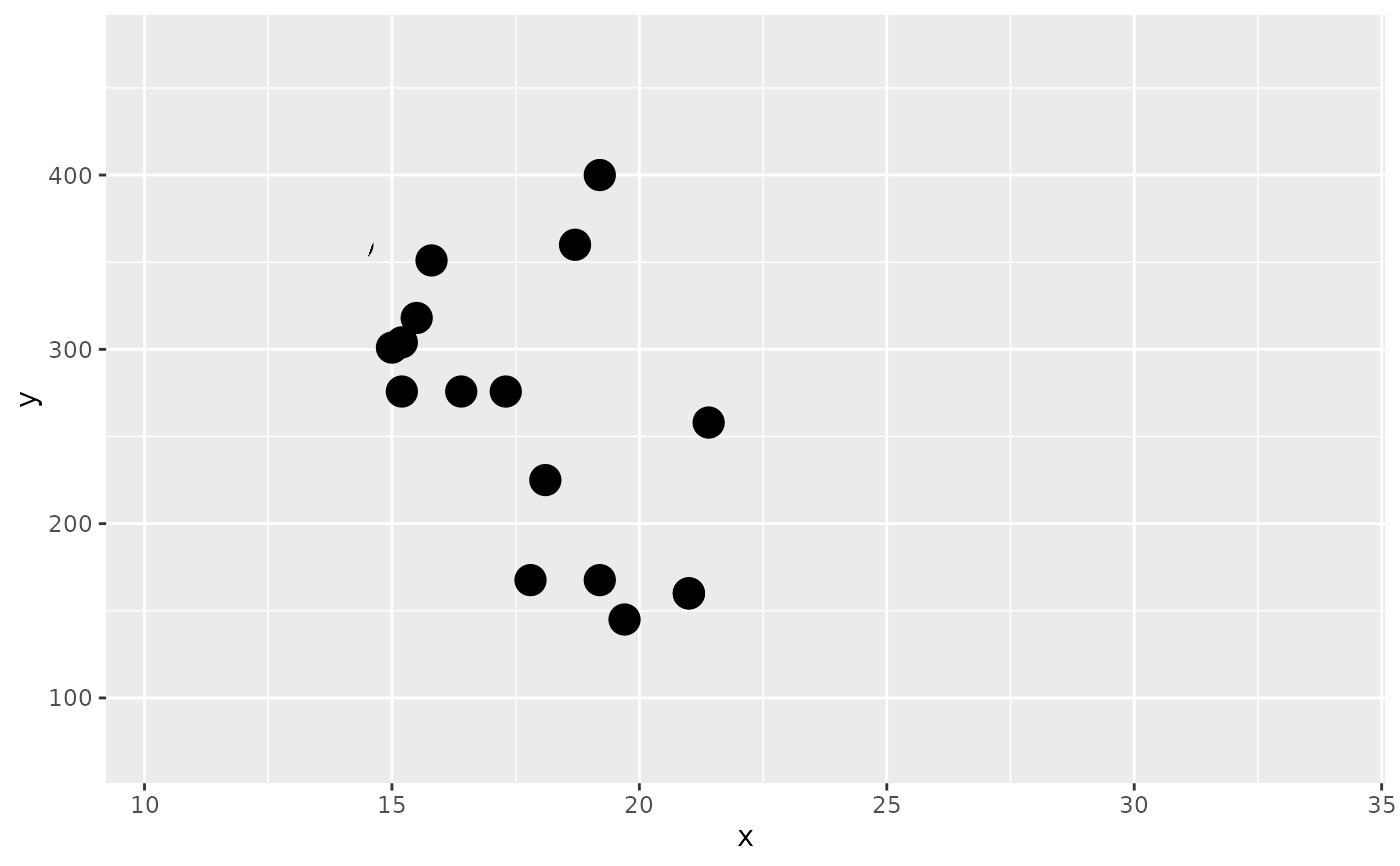This function is basically synonymous with with_raster() but exist to make the intend of marking a layer with a specific id clear.

as_reference(x, id = NULL, include = is.null(id))

## Arguments

x A ggplot2 layer object, a ggplot, a grob, or a character string naming a filter A string identifying this layer for later use Should the layer itself be included in the graphic

## Value

Depending on the input, either a grob, Layer, list of Layers, guide, or element object. Assume the output can be used in the same context as the input.

## See also

Other layer references: as_colourspace(), as_group()

## Examples

library(ggplot2)

ggplot() +
as_reference(
geom_point(aes(20, 300), size = 100, colour = 'white'),
id = 'mask_layer'
) +
with_mask(
geom_point(aes(mpg, disp), mtcars, size = 5),
mask = 'mask_layer'
)